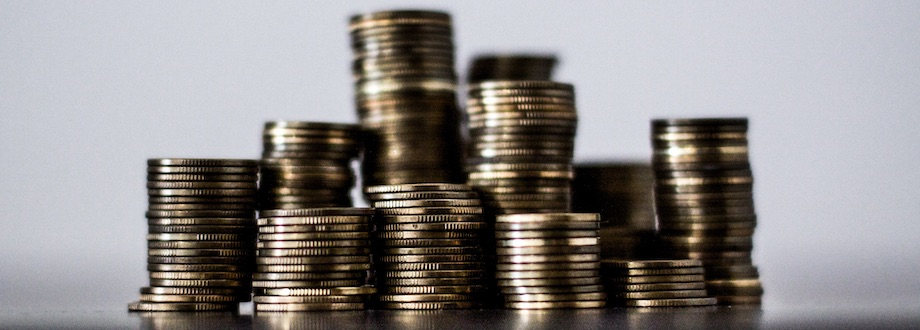# What is Recurring Deposit?

MoneyRecurring Deposit or RD is an investment option offered by banks where we deposit a certain sum of money every month for a fixed number of months at a given rate of interest.

A Recurring Deposit account is different from a Fixed Deposit account. In an FD account, we invest a lump sum and wait for it to mature. So, the FD account earns interest throughout its investment period.

## Why open a Recurring Deposit account?

If you don't have a lump sum to invest but can surely save a certain amount of money every month then go for Recurring Deposit.

For example, you can start a Recurring Deposit account for 12 months if you are sure that you can save INR 10,000 every month for the next 12 months.

## Is Recurring Deposit account taxable?

Yes. Your bank may deduct tax, if applicable, on the interest you earn. Do check the bank Recurring Deposit terms and conditions for more details.

## Which has the higher return? Recurring Deposit or Fixed Deposit?

Fixed Deposit accounts generally earn higher interest than a Recurring Deposit account.

For example, let's say Tom opened a Fixed Deposit account with INR 120,000. He invests the money for 12 months at 7% per annum interest rate.

He also opens a Recurring Deposit account and puts INR 10,000 every month for 12 months at the same interest rate.

As most banks will calculate interest compounded quarterly, we will get the following results.

### Return from Fixed Deposit account.

 Principal ₹ 120,000 Interest ₹ 8,623.08 Amount ₹ 128,623.08 Effective Yield 7.1859%

### Return from Recurring Deposit account.

 Total Investment ₹ 120,000 Interest ₹ 4,621.32 Amount ₹ 124,621.32 Effective Yield 3.8511%

As we can see in the above tables, the return from Fixed Deposit is higher compared to Recurring Deposit even though the same amount is invested for the same time period at the same rate of interest.

## Points to remember

• You can invest a fixed amount of money every month in a Recurring Deposit account.
• Interest earned is taxable.
• Recurring Deposit account earns lower interest than Fixed Deposit account for same amount, time period and rate of interest.
• Good option if you don't have a lump sum to invest in FD but can save a certain amount every month.
• Risk free investment as return is certain.

Check out this Recurring Deposit Calculator to calculate recurring deposit returns.